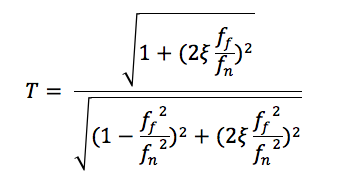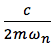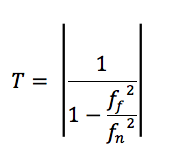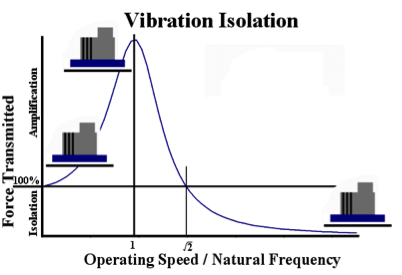# How Well Does a Vibration Isolator Work?

The proportion of vibrational energy transmitted by a vibration Isolator is given by:Where:

ff = forcing frequency of vibration (Hz)
fn = natural frequency of vibration isolator (Hz)

ξ == critical damping ratio (typical values for steel 0.005 – 0.01; rubber 0.05 – 0.1)

m = mass (kg)
c = viscous damping (Nsm-1)
ωn = 2 π fn

For lightly damped materials, such as steel springs, the critical damping ratio is negligible. The formula can then be simplified to:This shows that when resonance occurs (ff= fn) T tends to infinity (i.e. there is significant amplification of the transmitted force rather than any isolation).For isolation to occur T must be less than one, which only occurs when:However, the actual performance of a vibration isolator can be compromised by several factors that must also be taken into account when designing or selecting a vibration Isolator [Find Out More].